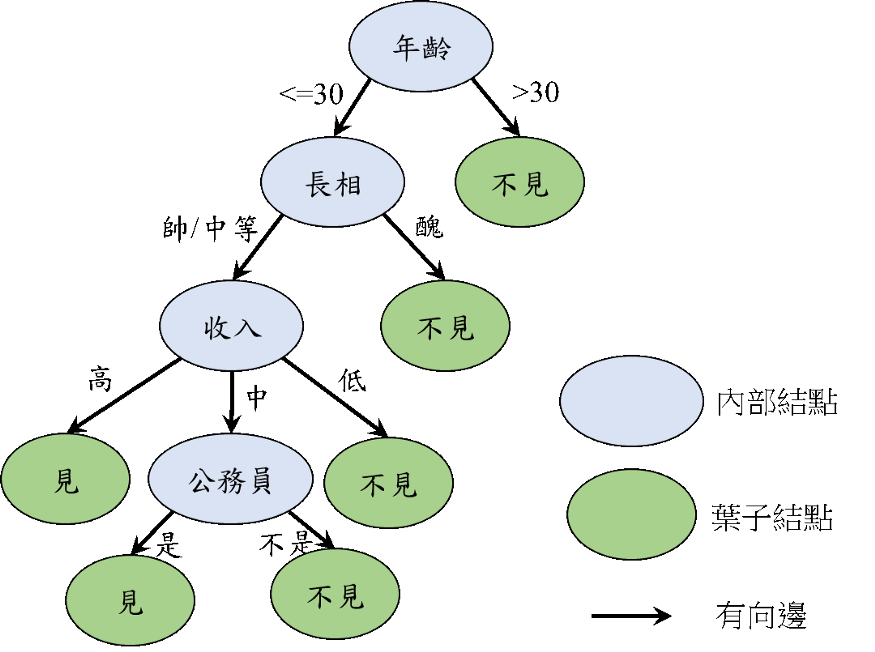#6

## 【用sklearn學習機器學習】快速學會套用sklearn模組，分類方法總覽-決策樹、隨機森林、SVM、KNN、羅吉斯回歸，學會看預測結果

sklearn是一個好用的機器學習套件，

scikei-learn版本: 0.20.1

# 主題- 分類

「讀取資料」->「將資料分成訓練集(training data)和測試集(testing data)」->
「呼叫sklearn的分類方法開始訓練分類模型」->「用訓練好的模型預測分類結果」

## 第一步:取得資料

``````from sklearn.datasets import load_iris
x_data, y_data = iris['data'], iris['target']
target_names = iris['target_names']
print(x_data)
print(y_data)
print(target_names)
``````

iris是鳶尾花資料，共150筆資料，
x_data是150*4的二維陣列，

y_data表示每筆資料對應的花的種類，有Setosa，Versicolor和Virginica三個品種
(可參考[資料分析&機器學習] 第2.1講： 如何獲取資料？ Sklearn內建資料集)

target_names就是把三種花的類別名稱取出來，即`['setosa' 'versicolor' 'virginica']`

## 第二步:將資料分成訓練集(training data)和測試集(testing data)

(若是較舊的版本可能要寫`from sklearn.cross_validation import train_test_split`)

``````from sklearn.model_selection import train_test_split
# from sklearn.cross_validation import train_test_split # for較舊的版本
x_train, x_test, y_train, y_test = train_test_split(x_data, y_data, test_size=0.2, random_state=41)
``````

train_test_split的參數涵義:

x_data: 鳶尾花的特徵資料
y_data: 鳶尾花的類別
test_size = 0.2 : 表示有80%的資料用來訓練，20%的資料結果測試
random_state: 隨機種子，設一樣的話資料分割的結果就一樣

• 訓練資料共120筆
• 測試資料共30筆

## 第三步:呼叫sklearn的分類方法開始訓練分類模型

sklearn有許多分類方法可以套用，

``````from sklearn.tree import DecisionTreeClassifier
model = DecisionTreeClassifier() #選擇一種分類算法
model.fit(x_train,y_train)  #用訓練資料訓練分類器
predictions = model.predict(x_test) #用分類器對測試資料做分類
``````

``````from sklearn.svm import SVC
model = SVC()               #選擇一種分類算法
model.fit(x_train,y_train)  #用訓練資料訓練分類器
predictions = model.predict(x_test) #用分類器對測試資料做分類
``````

## 第四步:用訓練好的模型預測分類結果

``````from sklearn.metrics import classification_report, confusion_matrix
print(confusion_matrix(y_test,predictions))
print(classification_report(y_test,predictions, target_names = target_names))
``````

``````[[ 9  0  0]
[ 0 10  1]
[ 0  2  8]]
precision    recall  f1-score   support

setosa       1.00      1.00      1.00         9
versicolor       0.83      0.91      0.87        11
virginica       0.89      0.80      0.84        10

micro avg       0.90      0.90      0.90        30
macro avg       0.91      0.90      0.90        30
weighted avg       0.90      0.90      0.90        30
``````

## 理解confusion_matrix

confusion_matrix以矩陣表示分類結果，

``````[[ 9  0  0]
[ 0 10  1]
[ 0  2  8]]
``````

(花的種類有Setosa，Versicolor和Virginica三個品種)

• 真實資料是Setosa、分類器也分類到Setosa的資料有9筆
• 真實資料是Versicolor、分類器也分類到Versicolor的資料有10筆
• 真實資料是Versicolor、分類器也分類到Virginica的資料有1筆
• 真實資料是Virginica、分類器也分類到Versicolor的資料有2筆
• 真實資料是Virginica、分類器也分類到Virginica的資料有8筆

## 理解precision, recall, f1-score

``````              precision    recall  f1-score   support

setosa       1.00      1.00      1.00         9
versicolor       0.83      0.91      0.87        11
virginica       0.89      0.80      0.84        10

micro avg       0.90      0.90      0.90        30
macro avg       0.91      0.90      0.90        30
weighted avg       0.90      0.90      0.90        30
``````

• precision是以預測結果出發，在機器說「你的分類是x類」時，說對的比率有多少
• recall又稱召回率，看「真實資料是x類」，機器真的把它分到x類的比率

(看直向資料)

10筆真的是versicolor、其它2筆判斷錯，

(看橫向資料)

f1-score是precision和recall的調和平均

## precision與recall的取捨

(分兩類的問題: 是「詐欺」類別，或者不是)

「詐欺」分類的precision高表示: 如果機器說你是詐欺，就高機率真的是詐欺(不會冤枉人，但有可能詐欺案發生但沒抓到)
「詐欺」分類的recall高表示: 如果真的發生詐欺案，機器抓出來的比率高(但有可能沒有詐欺也會被冤枉詐欺)

1. 如果銀行希望不要冤枉人，必須非常確定「詐欺」發生才抓，那就希望precision高
2. 如果銀行認為每次發生「詐欺案」會造成銀行的巨大損失，寧錯抓也不縱放，就希望recall高

# 完整程式碼範例

``````# 第一步:取得資料
x_data, y_data = iris['data'], iris['target']
target_names = iris['target_names']

# 第二步:將資料分成訓練集(training data)和測試集(testing data)
from sklearn.model_selection import train_test_split
# from sklearn.cross_validation import train_test_split # for較舊的版本
x_train, x_test, y_train, y_test = train_test_split(x_data, y_data, test_size=0.2, random_state=41)

# 第三步:呼叫sklearn的分類方法開始訓練分類模型
from sklearn.tree import DecisionTreeClassifier
model = DecisionTreeClassifier() #選擇一種分類算法
model.fit(x_train,y_train)  #用訓練資料訓練分類器
predictions = model.predict(x_test) #用分類器對測試資料做分類

# 第四步:用訓練好的模型預測分類結果
from sklearn.metrics import classification_report, confusion_matrix
print(confusion_matrix(y_test,predictions))
print(classification_report(y_test,predictions, target_names = target_names))
``````

# 分類方法總覽- 決策樹、隨機森林、SVM(支持向量機)、KNN(k近鄰算法)、羅吉斯回歸

``````from sklearn.tree import DecisionTreeClassifier # 決策樹
from sklearn.ensemble import RandomForestClassifier # 隨機森林
from sklearn.svm import SVC #支持向量機
from sklearn.neighbors import KNeighborsClassifier # k近鄰算法
from sklearn.linear_model import LogisticRegression # 羅吉斯回歸
``````

## 決策樹(Decision trees)

Decision trees（決策樹）是一種過程直覺單純、執行效率也相當高的監督式機器學習模型，適用於classification及regression資料類型的預測，與其它的ML模型比較起來，執行速度是它的一大優勢。(圖片來源: 機器學習: 決策樹 (Decision Tree))

1